Pythagoras' Theorem Exercise

A self marking exercise on the application of Pythagoras' Theorem.

Level 1Level 2Level 3Level 4Exam-StyleThree DDescriptionHelpMore Pythagoras

Here are some questions which can be answered using Pythagoras' Theorem. You can earn a trophy if you get at least 14 questions correct. Each time you finish a question click the 'Check' button lower down the page to see if you got it right!
[Don't forget to include the units in your answers after question one]

 1. What is the name for the longest side of a right angled triangle?2. What is the length of the longest side of a right angled triangle if the two shorter sides are 3cm and 4cm?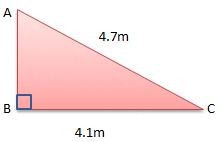3. Find the length of AB to 1 decimal place.4. Find the length of EG to 1 decimal place.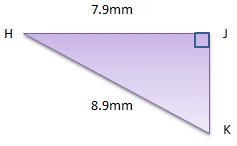5. Find the length of JK to 1 decimal place.6. A rectangular swimming pool is 23m wide and 46m long. Calculate the length of a diagonal in metres to 1 decimal place.7. A ladder is 6m long. How far from the base of a wall should it be placed if it is to reach 5m up the wall? Give your answer in metres correct to 1 decimal place8. A tent guy line supports one of the upright tent poles. It runs from the top of the pole to a peg in the ground two and a half metres away from the base of the pole. If the guy line is 427cm long, how tall is the upright tent pole? Give your answer in centimetres correct to the nearest centimetre.9. How long is the diagonal of an A4 size sheet of paper? The dimensions of A4 paper are 210 by 297 millimetres (8.3 inches × 11.7 inches). Give your answer in cm to one decimal place.10. For international matches football pitches must be of regulation size. The goal lines must be between 64 and 75 metres (70 and 80 yards) long and the touchlines must be between 100 and 110 metres (110 and 120 yards).What is the difference between the length of the diagonal of the largest acceptable pitch and the length of the diagonal of the smallest acceptable pitch? Give your answer in metres to the nearest metre.11. Find the length of a side of a rhombus whose diagonals are of length 13km and 17km. Give your answer in kilometers correct to one decimal place.3cm9cm10cm12. Find the perimeter of this parallelogram to one decimal place.13. The length of the diagonal of a square is 60m. How long are the sides of the square? Give your answer correct to one decimal place.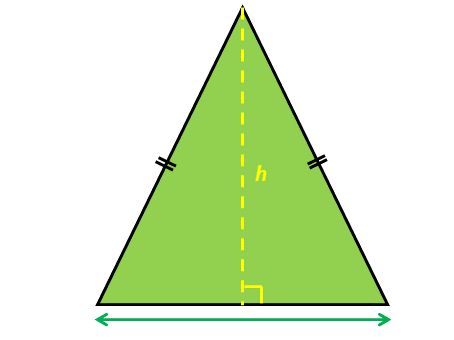13cm15cm14. Find the height (h) of this isosceles triangle to one decimal place.15. The sign says 'Keep off the grass'. Each day Michael has to get from one corner of the rectangular area of grass to the opposite corner. If the park keeper is looking he will walk along the edges but if the park keeper is not looking he will take the direct route, diagonally across the rectangle.How much further does Michael walk on the days when the park keeper is looking? The length of the rectangular area of grass is 160m and the width is 107m. Give your answer to the nearest metre.16. The blue squares have sides of length 32mm and the red square has sides of length 43mm. Find the distance from A to B in centimetres correct to one decimal place.17. An irregular quadrilateral ABCD has right angles at the opposite vertices A and C. Calculate the length of the side DA if AB=44.1cm, BC=44.3cm and CD=46.1cm. Give your answers in cm to one decimal place.18. An aeroplane flies due north for 296km then changes direction and flies east for 415km. How far is it now in a straight line from its starting position. Give your answer to the nearest kilometre.19. A ship sails on a bearing of 045o for 263km then changes direction and sails on a bearing of 135o for 427km. Finally it then turns and sails for 60km on a bearing of 225o. How far is it now in a straight line from its starting position. Give your answer to the nearest kilometre.20. One side of a right angled triangle is 10cm. The other two sides are both of length x. Calculate x to 3 significant figures.Check

This is Pythagoras' Theorem Exercise level 4. You can also try:
Level 1 Level 2 Level 3

Instructions

Try your best to answer the questions above. Type your answers into the boxes provided leaving no spaces. As you work through the exercise regularly click the "check" button. If you have any wrong answers, do your best to do corrections but if there is anything you don't understand, please ask your teacher for help.

When you have got all of the questions correct you may want to print out this page and paste it into your exercise book. If you keep your work in an ePortfolio you could take a screen shot of your answers and paste that into your Maths file.

Transum.org

This web site contains over a thousand free mathematical activities for teachers and pupils. Click here to go to the main page which links to all of the resources available.More Activities:

Mathematicians are not the people who find Maths easy; they are the people who enjoy how mystifying, puzzling and hard it is. Are you a mathematician?

Comment recorded on the 17 June 'Starter of the Day' page by Mr Hall, Light Hall School, Solihull:

"Dear Transum,

I love you website I use it every maths lesson I have with every year group! I don't know were I would turn to with out you!"

Comment recorded on the 11 January 'Starter of the Day' page by S Johnson, The King John School:

"We recently had an afternoon on accelerated learning.This linked really well and prompted a discussion about learning styles and short term memory."

Digital Darts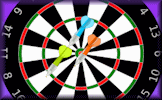An online darts game for one or two players requiring skill, strategy and mental arithmetic. It provides an enjoyable way to practise adding and subtracting two and three digit numbers.

There are answers to this exercise but they are available in this space to teachers, tutors and parents who have logged in to their Transum subscription on this computer.

A Transum subscription unlocks the answers to the online exercises, quizzes and puzzles. It also provides the teacher with access to quality external links on each of the Transum Topic pages and the facility to add to the collection themselves.

Subscribers can manage class lists, lesson plans and assessment data in the Class Admin application and have access to reports of the Transum Trophies earned by class members.

Subscribe

Go Maths

Learning and understanding Mathematics, at every level, requires learner engagement. Mathematics is not a spectator sport. Sometimes traditional teaching fails to actively involve students. One way to address the problem is through the use of interactive activities and this web site provides many of those. The Go Maths page is an alphabetical list of free activities designed for students in Secondary/High school.

Maths Map

Are you looking for something specific? An exercise to supplement the topic you are studying at school at the moment perhaps. Navigate using our Maths Map to find exercises, puzzles and Maths lesson starters grouped by topic.

Teachers

If you found this activity useful don't forget to record it in your scheme of work or learning management system. The short URL, ready to be copied and pasted, is as follows:

Do you have any comments? It is always useful to receive feedback and helps make this free resource even more useful for those learning Mathematics anywhere in the world. Click here to enter your comments.For All:

Description of Levels

CloseLevel 1 - Finding the hypotenuse

Level 2 - Finding a shorter side

Level 3 - Mixed questions

Level 4 - Pythagoras' Theorem exercise

Exam Style questions requiring an application of Pythagoras' Theorem and trigonometric ratios to find angles and lengths in right-angled triangles.

Three Dimensions - Three dimensional Pythagoras and trigonometry questions

More on this topic including lesson Starters, visual aids, investigations and self-marking exercises.

Answers to this exercise are available lower down this page when you are logged in to your Transum account. If you don’t yet have a Transum subscription one can be very quickly set up if you are a teacher, tutor or parent.

Curriculum Reference

See the National Curriculum page for links to related online activities and resources.

Pythagoras' Theorem

The area of the square on the hypotenuse of a right angled triangle is equal to the sum of the areas of the squares on the two shorter sides.You may have learned the theorem using letters to stand for the lengths of the sides. The corners (vertices) of the right-angled triangle is labelled with capital (upper case) letters. The lengths of the sides opposite them are labelled with the corresponding small (lower case) letters.Alternatively the sides of the right-angled triangle may me named using the capital letters of the two points they span.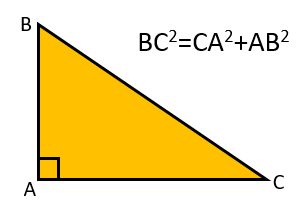As triangle can be labelled in many different ways it is probably best to remember the theorem by momorising the first diagram above.

To find the longest side (hypotenuse) of a right-angled triangle you square the two shorter sides, add together the results and then find the square root of this total.

To find a shorter side of a right-angled triangle you subtract the square of the other shorter side from the square of the hypotenuse and then find the square root of the answer.

ExampleAB2 = AC2 - BC2
AB2 = 4.72 - 4.12
AB2 = 22.09 - 16.81
AB2 = 5.28
AB = √5.28
AB = 2.3m (to one decimal place)

The diagrams aren't always the same way round. They could be rotated by any angle.The right-angled triangles could be long and thin or short and not so thin.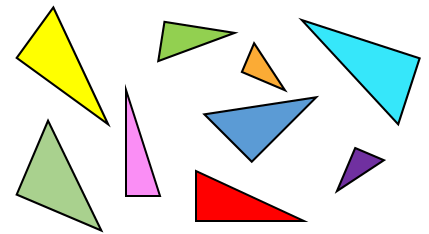So why is Pythagoras' theorem true?

The video above is from Australia's most unlikely new celebrity, Eddie Woo.

Don't wait until you have finished the exercise before you click on the 'Check' button. Click it often as you work through the questions to see if you are answering them correctly.

Close